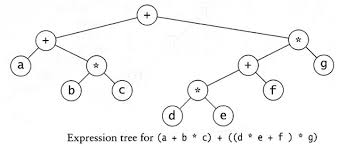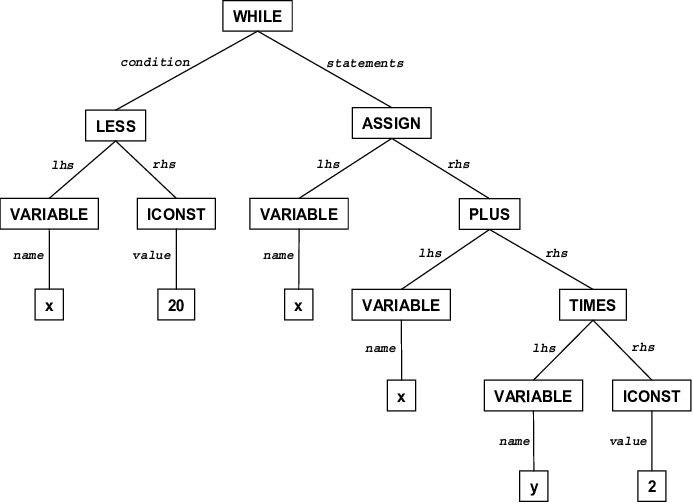# Applications of Binary TreesCreated August 4, 2021

So far we've discussed about how we can perform certain operations on a Binary Search Tree. It is no fun to learn about new stuff when you don't know where exactly you can use it. So, In this article I'm gonna be talking about the applications of Binary Tree Data Structure.

But first, lets find the answer to a simple question : Why should we use a binary tree? What's the need to use a Binary Search Tree? Because we already know of many other data structures that can store the same amount of data and that too in a less complex way. An array can be a good example for that.

## Why should we use a Binary Search Tree?

Well, there are many reasons for why you should use a binary tree. Some are as follows:

1. Data is stored level by level in a hierarchal order. So, visualising the stored data becomes easier.
2. Data is stored in an ordered manner. So searching becomes easier. Also, the time complexity for searching in a BST is much less than that of a simple array.
3. Sorting the data also gets easier as the data is already present in a somewhat sorted order. So, BSTs are mostly advantageous for sorting and searching operations.

## Applications

### Expression Trees

In this application, consider a simple expression. The leaves of the tree denote the operands and the rest of the nodes denote the operators.### In Compilers

The IDE(Integrated Development Environment) in which you write your code primarily consists of three parts - the source code editor(where you write your code), compiler/interpreter(a program that builds the code before running it) and a debugger(which checks the errors in the code). A compiler is nothing but a program that translates the code you write in the editor (also known as "High Level Language") in the form that the system or your computer can understand (also known as "Low Level Language").

For instance, C is a compiled language. That means when you run your C code, it is first compiled and then executed.

So, in this compilation process, compilers use something called a "Syntax Tree" to efficiently carry out the process. It looks something like this -### In Huffman Coding Algorithm

Huffman coding algorithm is used for data compression. It generates the Huffman Code for a given message based on the frequency of each character present in that message.

This algorithm makes use of a Binary Tree called "Huffman coding tree" or "Huffman tree". It is a frequency sorted Binary Tree to to encode all the characters.

### In Unix Kernel

Virtual Memory is a part of computer's secondary memory which acts as a part of it's primary memory(or RAM). While running bulky applications, some RAM data is shifted to Virtual Memory in order to avoid overloading on RAM so that the process can work efficiently.

BSTs are used in managing a set of these Virtual Memory Areas or VMAs.

There can be many other uses of Binary Search Trees. As we previously discussed, BSTs are widely used in sorting algorithms. Also, it makes searching an easy process.

## That's it

This is it for this one. You can check out my other posts here. Thanks for reading 😊

Happy learning!